# JS 系列二：深入 constructor、prototype、__proto__、[[Prototype]] 及 原型链

### 引言

`JS`系列暂定 27 篇，从基础，到原型，到异步，到设计模式，到架构模式等，

### 一、基础入门

#### 1. 对象

``````let obj1 = {};
let obj2 = new Object();
let obj3 = new fun1()

function fun1(){};
let fun2 = function(){};
let fun3 = new Function('some','console.log(some)');

// JS自带的函数对象
console.log(typeof Object); //function
console.log(typeof Function); //function

// 普通对象
console.log(typeof obj1); //object
console.log(typeof obj2); //object
console.log(typeof obj3); //object

// 函数对象
console.log(typeof fun1); //function
console.log(typeof fun2); //function
console.log(typeof fun3); //function

#### 2. 构造函数

``````function Foo(name, age) {
// this 指向 Foo
this.name = name
this.age = age
this.class = 'class'
// return this // 默认有这一行
}

// Foo 的实例
let f = new Foo('aa', 20)

``````f.constructor === Foo // true

JS 本身不提供一个 `class` 实现。（在 ES2015/ES6 中引入了 `class` 关键字，但只是语法糖，JavaScript 仍然是基于原型的）。

#### 3. 构造函数扩展

• `let a = {}` 其实是 `let a = new Object()` 的语法糖
• `let a = []` 其实是 `let a = new Array()` 的语法糖
• `function Foo(){ ... }` 其实是 `var Foo = new Function(...)`
• 可以使用 `instanceof` 判断一个函数是否为一个变量的构造函数

#### 4. Symbol 是构造函数吗？

Symbol 是基本数据类型，它并不是构造函数，因为它不支持 `new Symbol()` 语法。我们直接使用`Symbol()` 即可。

``````let an = Symbol("An");

let an1 = new Symbol("An");
// Uncaught TypeError: Symbol is not a constructor

``````Symbol("An").constructor;
// ƒ Symbol() { [native code] }

``````Symbol.prototype.constructor;
// ƒ Symbol() { [native code] }

##### `Symbol()` 返回的 symbol 值是唯一的
``````Symbol("An") === Symbol("An");
// false

##### 可以通过 `Symbol.for(key)` 获取全局唯一的 symbol
``````Symbol.for('An') === Symbol.for("An"); // true

##### Symbol.iterator ：返回一个对象的迭代器
``````// 实现可迭代协议，使迭代器可迭代：Symbol.iterator
function createIterator(items) {
let i = 0
return {
next: function () {
let done = (i >= items.length)
let value = !done ? items[i++] : undefined
return {
done: done,
value: value
}
},
[Symbol.iterator]: function () {
return this
}
}
}
const iterator = createIterator([1, 2, 3]);
[...iterator];		// [1, 2, 3]

##### Symbol.toPrimitive：将对象转换成基本数据类型
``````// Symbol.toPrimitive 来实现拆箱操作（ES6 之后）
let obj = {
valueOf: () => {console.log("valueOf"); return {}},
toString: () => {console.log("toString"); return {}}
}
obj[Symbol.toPrimitive] = () => {console.log("toPrimitive"); return "hello"}
console.log(obj + "")
// toPrimitive
// hello

##### Symbol.toStringTag：用于设置对象的默认描述字符串值
``````// Symbol.toStringTag 代替 [[class]] 属性（ES5开始）
let o = { [Symbol.toStringTag]: "MyObject" }

console.log(o + "");
// [object MyObject]

#### 5. constructor 的值是只读的吗？

##### 引用类型
``````function An() {
this.value = "An";
};
function Anran() {};

Anran.prototype.constructor = An;
// 原型链继承中，对 constructor 重新赋值

let anran = new Anran();
// 创建 Anran 的一个新实例

console.log(anran);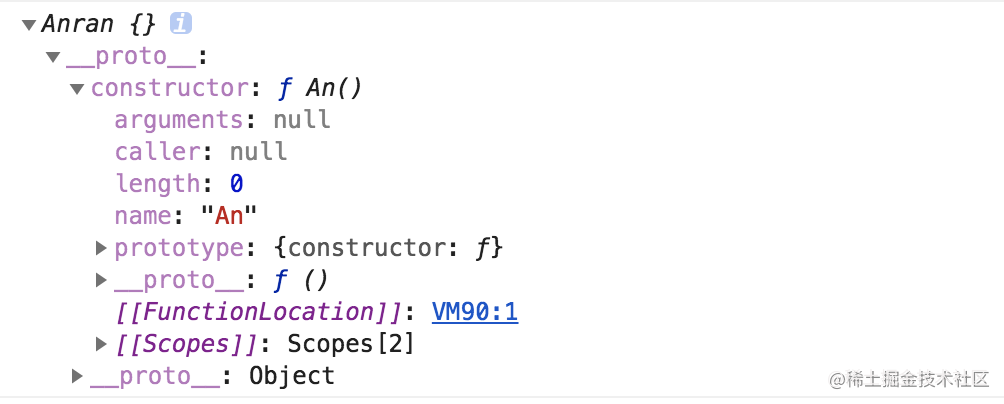##### 基本类型
``````function An() {};
let an = 1;
an.constructor = An;
console.log(an.constructor);
// ƒ Number() { [native code] }

JS 对于不可写的属性值的修改静默失败（silently failed），但只会在严格模式下才会提示错误。

``````'use strict';
function An() {};
let an = 1;
an.constructor = An;
console.log(an.constructor);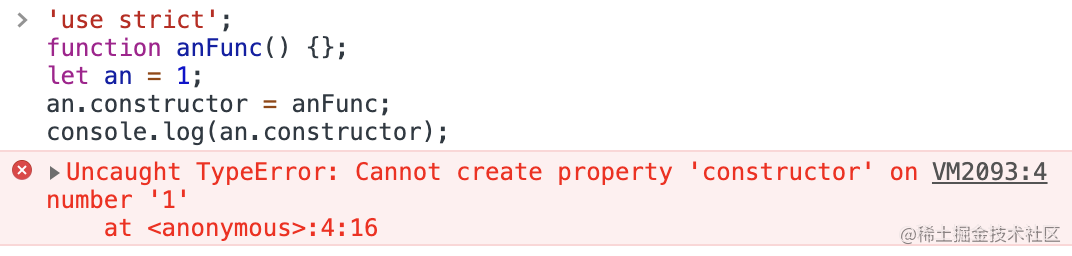### 二、原型

``````// 构造函数
function Foo(name) {
this.name = name
}
}
// 创建实例
let f = new Foo('some')
f.printName = function () {
console.log(this.name)
}
// 测试
f.printName()// 对象的方法

#### 1. prototype

``````let fun = Function.prototype.bind();
// ƒ () { [native code] }

``````function Foo () {}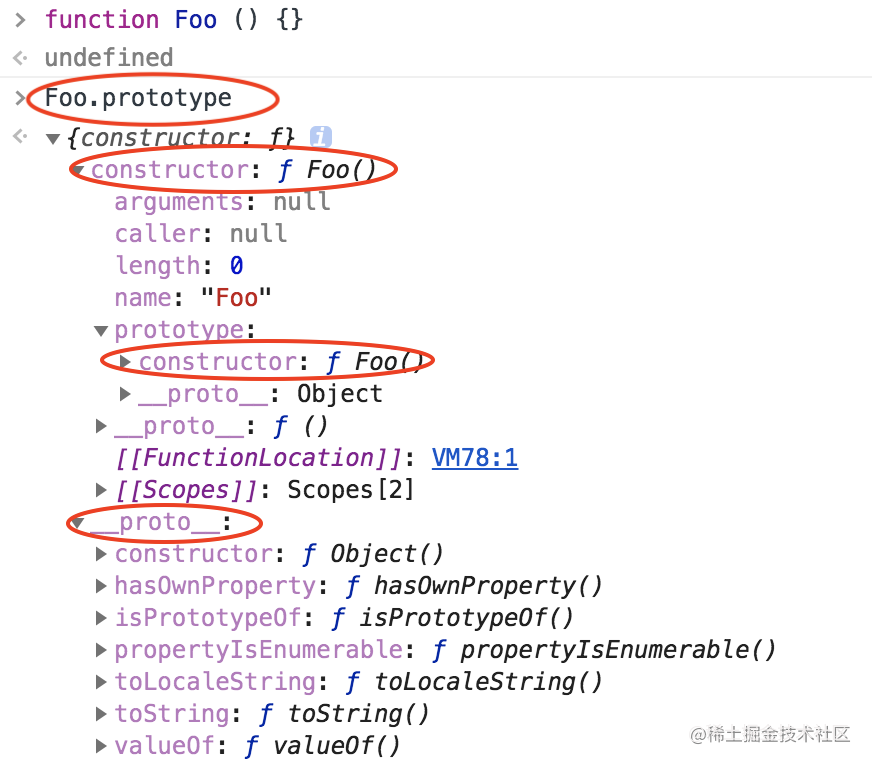`prototype` 属性就被自动创建了

``````Foo.prototype.constructor === Foo; // true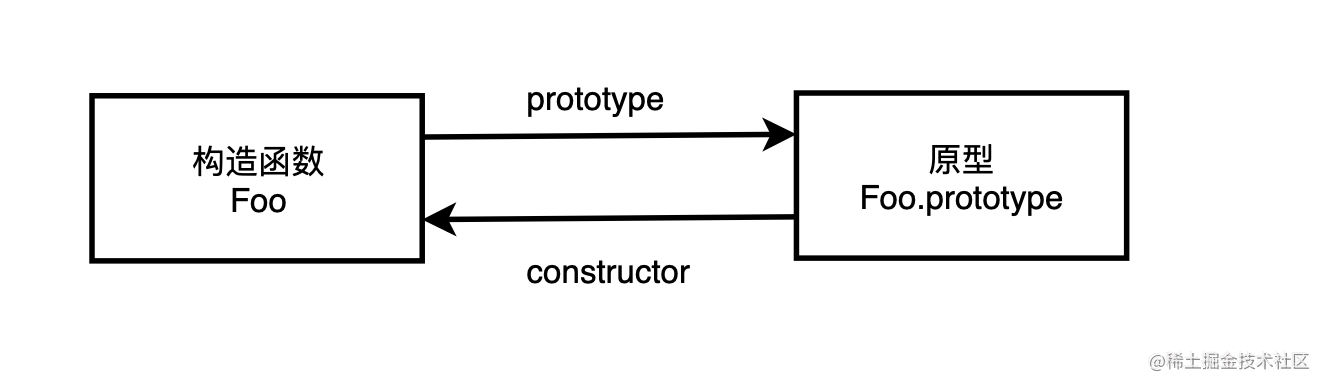#### 2. `__proto__`

``````function Foo () {}
let foo = new Foo()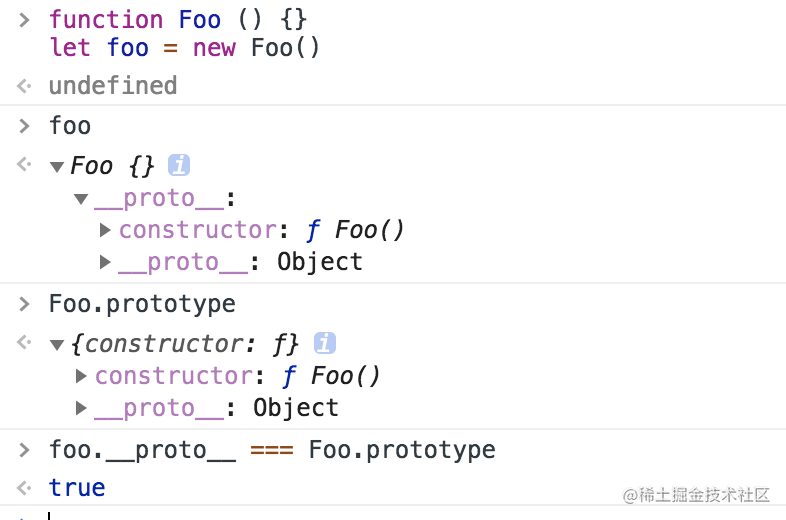`new Foo()` 时，`__proto__` 被自动创建。并且

``````foo.__proto__ === Foo.prototype; // true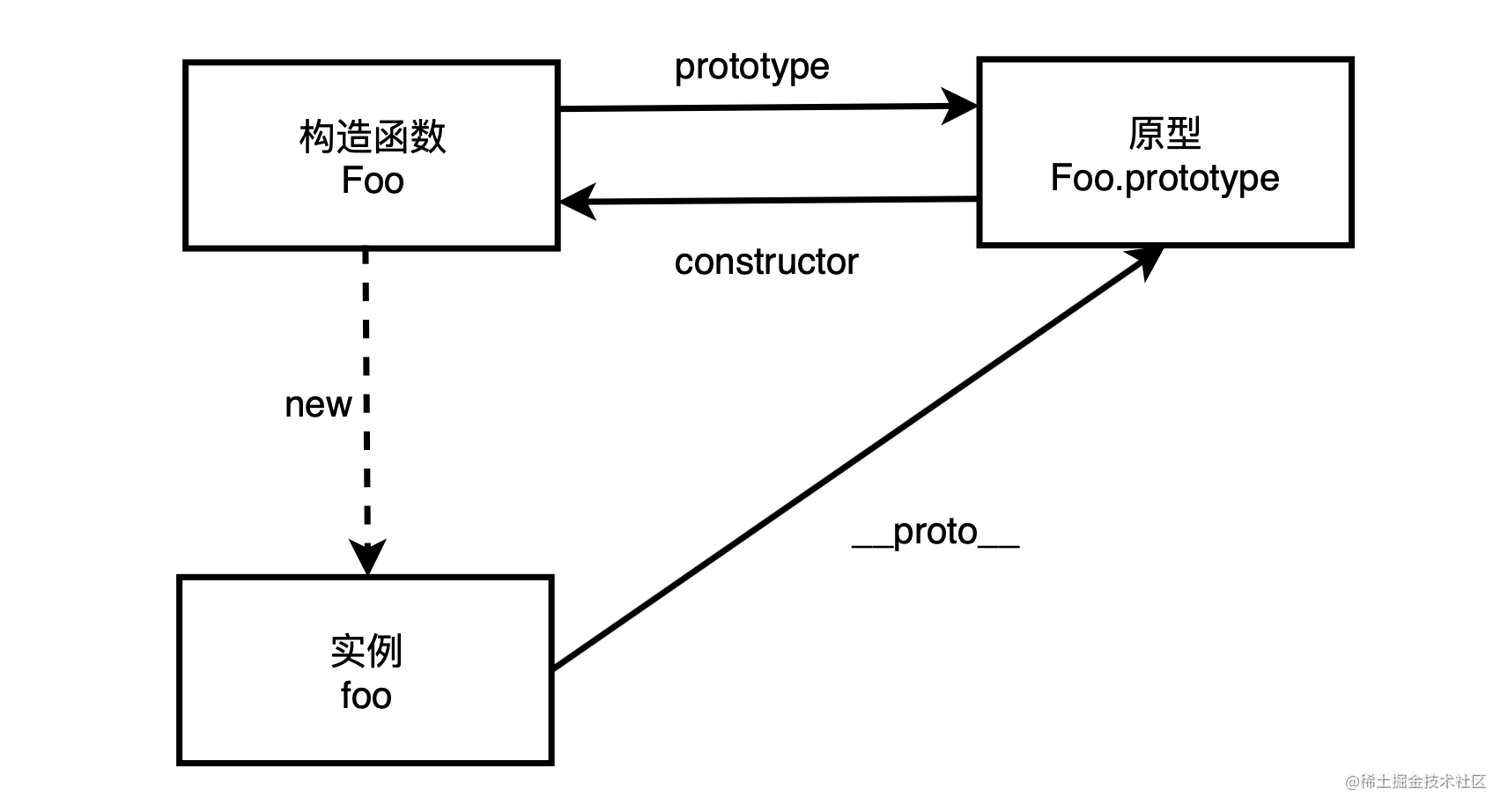`__proto__` 发音 dunder proto，最先被 Firefox使用，后来在 ES6 被列为 Javascript 的标准内建属性。

#### 3. [[Prototype]]

`[[Prototype]]` 是对象的一个内部属性，外部代码无法直接访问。

#### 4. 注意

`__proto__` 属性在 `ES6` 时才被标准化，以确保 Web 浏览器的兼容性，但是不推荐使用，除了标准化的原因之外还有性能问题。为了更好的支持，推荐使用 `Object.getPrototypeOf()`

``````// 获取（两者一致）
Object.getPrototypeOf()
Reflect.getPrototypeOf()

// 修改（两者一致）
Object.setPrototypeOf()
Reflect.setPrototypeOf()

``````function An() {};
var an = new An();
var anran = Object.create(an);

#### 5. new 的实现过程

• 新生成了一个对象

• 链接到原型

• 绑定 this

• 返回新对象

``````function new_object() {
// 创建一个空的对象
let obj = new Object()
// 获得构造函数
let Con = [].shift.call(arguments)
// 链接到原型 （不推荐使用）
obj.__proto__ = Con.prototype
// 绑定 this，执行构造函数
let result = Con.apply(obj, arguments)
// 确保 new 出来的是个对象
return typeof result === 'object' ? result : obj
}

##### 优化 new 实现
``````// 优化后 new 实现
function create() {
// 1、获得构造函数，同时删除 arguments 中第一个参数
Con = [].shift.call(arguments);
// 2、创建一个空的对象并链接到原型，obj 可以访问构造函数原型中的属性
let obj = Object.create(Con.prototype);
// 3、绑定 this 实现继承，obj 可以访问到构造函数中的属性
let ret = Con.apply(obj, arguments);
// 4、优先返回构造函数返回的对象
return ret instanceof Object ? ret : obj;
};

#### 6. 总结

• 所有的引用类型（数组、对象、函数）都有对象特性，即可自由扩展属性（null除外）。
• 所有的引用类型，都有一个 `__proto__` 属性，属性值是一个普通的对象，该原型对象也有一个自己的原型对象(`__proto__`) ，层层向上直到一个对象的原型对象为 `null`。根据定义，`null` 没有原型，并作为这个原型链 中的最后一个环节。
• 当试图得到一个对象的某个属性时，如果这个对象本身没有这个属性，那么会去它的 `__proto__` （即它的构造函数的 `prototype` ）中寻找。

### 三、原型链

``````// 构造函数
function Foo(name) {
this.name = name
}
// 创建实例
let f = new Foo('some')
// 测试
f.toString()
// f.__proto__.__proto__中寻找

`f.__proto__=== Foo.prototype``Foo.prototype` 也是一个对象，也有自己的`__proto__` 指向 `Object.prototype`， 找到`toString()`方法。

``````Function.__proto__.__proto__ === Object.prototype

``````function Elem(id) {
this.elem = document.getElementById(id)
}

Elem.prototype.html = function(val) {
let elem = this.elem
if (val) {
elem.innerHTML = val
return this // 链式操作
} else {
return elem.innerHTML
}
}

Elem.prototype.on = function( type, fn) {
let elem = this.elem
}

let div1 = new Elem('div1')
// console.log(div1.html())
div1.html('<p>hello</p>').on('click', function() {
})// 链式操作

### 四、总结

• `Symbol` 是基本数据类型，并不是构造函数，因为它不支持语法 `new Symbol()`，但其原型上拥有 `constructor` 属性，即 `Symbol.prototype.constructor`
• 引用类型 `constructor` 是可以修改的，但对于基本类型来说它是只读的， `null``undefined` 没有 `constructor` 属性。
• `__proto__` 是每个实例对象都有的属性，`prototype` 是其构造函数的属性，在实例上并不存在，所以这两个并不一样，但 `foo.__proto__``Foo.prototype` 指向同一个对象。
• `__proto__` 属性在 `ES6` 时被标准化，但因为性能问题并不推荐使用，推荐使用 `Object.getPrototypeOf()`
• 每个对象拥有一个原型对象，通过 `__proto__` 指针指向上一个原型 ，并从中继承方法和属性，同时原型对象也可能拥有原型，这样一层一层向上，最终指向 `null`，这就是原型链。
• 当试图得到一个对象的某个属性时，如果这个对象本身没有这个属性，那么会去它的原型中寻找，以及该对象的原型的原型，一层一层向上查找，直到找到一个名字匹配的属性 / 方法或到达原型链的末尾（`null`

### 走在最后

1. 如有任何问题或更独特的见解，欢迎评论或直接联系瓶子君（公众号回复 123 即可）！

2. 欢迎关注：前端瓶子君，每日更新！